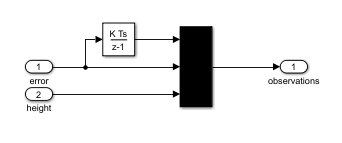# Water Tank Reinforcement Learning Environment Model

This example shows how to create a water tank reinforcement learning Simulink® environment that contains an RL Agent block in the place of a controller for the water level in a tank. To simulate this environment, you must create an agent and specify that agent in the RL Agent block. For an example that trains an agent using this environment, see Create Simulink Environment and Train Agent.

```mdl = 'rlwatertank'; open_system(mdl)```This model already contains an RL Agent block, which connects to the following signals:

• Scalar action output signal

• Vector of observation input signals

• Scalar reward input signal

• Logical input signal for stopping the simulation

### Actions and Observations

A reinforcement learning environment receives action signals from the agent and generates observation signals in response to these actions. To create and train an agent, you must create action and observation specification objects.

The action signal for this environment is the flow rate control signal that is sent to the plant. To create a specification object for an action channel carrying a continuous signal, use the `rlNumericSpec` function.

```actionInfo = rlNumericSpec([1 1]); actionInfo.Name = 'flow';```

If the action signal takes one of a discrete set of possible values, create the specification using the `rlFiniteSetSpec` function.

For this environment, there are three observation signals sent to the agent, specified as a vector signal. The observation vector is ${\left[\begin{array}{ccc}\int \mathit{e}\text{\hspace{0.17em}}\mathrm{dt}& \mathit{e}& \mathit{h}\end{array}\right]}^{\mathit{T}\text{\hspace{0.17em}}}$, where:

• $\mathit{h}$ is the height of the water in the tank.

• $\mathit{e}=\mathit{r}-\mathit{h}$, where $\mathit{r}$ is the reference value for the water height.

Compute the observation signals in the generate observations subsystem.

`open_system([mdl '/generate observations'])`Create a three-element vector of observation specifications. Specify a lower bound of 0 for the water height, leaving the other observation signals unbounded.

```observationInfo = rlNumericSpec([3 1],... 'LowerLimit',[-inf -inf 0 ]',... 'UpperLimit',[ inf inf inf]'); observationInfo.Name = 'observations'; observationInfo.Description = 'integrated error, error, and measured height';```

If the actions or observations are represented by bus signals, create specifications using the `bus2RLSpec` function.

### Reward Signal

Construct a scalar reward signal. For this example, specify the following reward.

`$\mathrm{reward}=10\left(|\mathit{e}|<0.1\right)-1\left(|\mathit{e}|\ge 0.1\right)-100\left(\mathit{h}\le 0||\mathit{h}\ge 20\right)$`

The reward is positive when the error is below `0.1` and negative otherwise. Also, there is a large reward penalty when the water height is outside the `0` to `20` range.

Construct this reward in the calculate reward subsystem.

`open_system([mdl '/calculate reward'])`### Stop Signal

To terminate training episodes and simulations, specify a logical signal to the `isdone` input port of the block. For this example, terminate the episode if $\mathit{h}\le 0$ or $\mathit{h}\ge 20$.

Compute this signal in the stop simulation subsystem.

`open_system([mdl '/stop simulation'])`### Create Environment Object

Create an environment object for the Simulink model.

`env = rlSimulinkEnv(mdl,[mdl '/RL Agent'],observationInfo,actionInfo);`

### Reset Function

You can also create a custom reset function that randomizes parameters, variables, or states of the model. In this example, the reset function randomizes the reference signal and the initial water height and sets the corresponding block parameters.

`env.ResetFcn = @(in)localResetFcn(in);`

#### Local Function

```function in = localResetFcn(in) % Randomize reference signal blk = sprintf('rlwatertank/Desired \nWater Level'); h = 3*randn + 10; while h <= 0 || h >= 20 h = 3*randn + 10; end in = setBlockParameter(in,blk,'Value',num2str(h)); % Randomize initial height h = 3*randn + 10; while h <= 0 || h >= 20 h = 3*randn + 10; end blk = 'rlwatertank/Water-Tank System/H'; in = setBlockParameter(in,blk,'InitialCondition',num2str(h)); end```